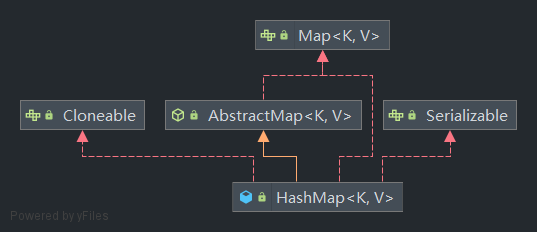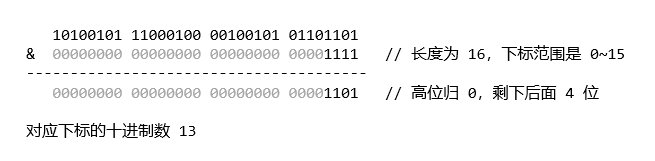# 基于 JDK 1.8 分析 HashMap 的底层原理

2021年5月31日 Oh! Java

Java 的 HashMap 可以说是用的最多、问的最多的一个 Map Collection 了。HashMap 是非同步的，即线程不安全。HashMap 允许存放的 key 为 null，但并不保证映射的顺序，也不保证这个顺序随时间保持不变。

``````public class HashMap<K,V> extends AbstractMap<K,V>
implements Map<K,V>, Cloneable, Serializable
``````## # 哈希表 + 链表 / 红黑树

``````static class Node<K,V> implements Map.Entry<K,V> {
final int hash;
final K key;
V value;
Node<K,V> next;
// ...
}

/**
* The table, initialized on first use, and resized as
* necessary. When allocated, length is always a power of two.
* (We also tolerate length zero in some operations to allow
* bootstrapping mechanics that are currently not needed.)
*/
transient Node<K,V>[] table;

/**
* The number of key-value mappings contained in this map.
*/
transient int size;
``````

HashMap 最基本的数据结构是哈希表 table（一个 Node 数组）。在 HashMap 中，把数组每个下标对应的空间称为 bucket（哈希桶），bucket 的数量就是数组的长度。

HashMap 的 table 是懒加载的，当我们创建 HashMap 实例的时候并不会马上创建 table 数组实例，而是在第一次向 HashMap 实例添加元素的时候，才会进行初始化。

``````public V put(K key, V value) {
return putVal(hash(key), key, value, false, true);
}

final V putVal(int hash, K key, V value, boolean onlyIfAbsent, boolean evict) {
Node<K,V>[] tab; Node<K,V> p; int n, i;
if ((tab = table) == null || (n = tab.length) == 0)
n = (tab = resize()).length; // 初始化 table
if ((p = tab[i = (n - 1) & hash]) == null)
tab[i] = newNode(hash, key, value, null);
else {
/* 发生哈希碰撞时的处理 */
}
// ...
return null;
}
``````

HashMap 存放和获取数据时，都需要通过计算 key 的 hash 值来并映射为 bucket 的下标。不同的 key 得到的下标可能相同，在存放新数据时，计算出来的下标对应的 bucket 中如果已经存在其它节点，这种情况称为哈希碰撞 / 哈希冲突

``````static final int TREEIFY_THRESHOLD = 8;

for (int binCount = 0; ; ++binCount) {
if ((e = p.next) == null) {
p.next = newNode(hash, key, value, null);
if (binCount >= TREEIFY_THRESHOLD - 1) // -1 for 1st
treeifyBin(tab, hash);
break;
}
if (e.hash == hash &&
((k = e.key) == key || (key != null && key.equals(k))))
break;
p = e;
}
``````

``````static final int MIN_TREEIFY_CAPACITY = 64;

final void treeifyBin(Node<K,V>[] tab, int hash) {
int n, index; Node<K,V> e;
if (tab == null || (n = tab.length) < MIN_TREEIFY_CAPACITY)
resize();
else if ((e = tab[index = (n - 1) & hash]) != null) {
// ...
}
}
``````

`MIN_TREEIFY_CAPACITY` 是 bucket 结构树形化 table 要求的最小容量，当 table 太小没有达到这个值时（固定是 64），HashMap 会采用扩容的方式来重新打散这些节点的分布。

• 当没有发生碰撞时，那么通过计算下标直接命中对应节点，时间复杂度为 O(1)
• 当下标位置是发生碰撞 bucket 时，如果起连接作用的数据结构是链表的话，那么时间复杂度为 O(n)，n 是链表长度
• 当链表过长转换为红黑树时，时间复杂度为 O(log(n))，n 是树的节点个数。

## # hash 与 下标计算

``````static final int hash(Object key) {
int h;
return (key == null) ? 0 : (h = key.hashCode()) ^ (h >>> 16);
}
``````HashMap 对这个扰乱操作是从实用性、速度、质量方面考虑的，关于这方面的说明，JDK 1.8 源码中一段注释我没完全看懂，这里贴在下面，或者可以自己去源码阅读完整的说明。

There is a tradeoff between speed, utility, and quality of bit-spreading. Because many common sets of hashes are already reasonably distributed (so don't benefit from spreading), and because we use trees to handle large sets of collisions in bins, we just XOR some shifted bits in the cheapest possible way to reduce systematic lossage, as well as to incorporate impact of the highest bits that would otherwise never be used in index calculations because of table bounds.

## # resize() 扩容机制

``````static final float DEFAULT_LOAD_FACTOR = 0.75f;

int threshold;

final V putVal(int hash, K key, V value, boolean onlyIfAbsent, boolean evict) {
// ...
if (++size > threshold)
resize();
afterNodeInsertion(evict);
return null;
}

public HashMap() {
}
``````

`loadFactor` 称为负载因子，默认是使用 `DEFAULT_LOAD_FACTOR` 的值 0.75，这代表在碰撞次数为 0 的情况下，有超过 75% 的 bucket 被使用就会进行扩容操作。0.75 是从时间和空间上进行权衡得出的值，当负载因子更大时，会减少空间的开销，因为扩容所要求的 `threshold` 会更高，但这也可能会增加查询的时间成本，因为每个 bucket 发生哈希冲突的次数和概率会变得更高。

``````/**
* The default initial capacity - MUST be a power of two.
*/
static final int DEFAULT_INITIAL_CAPACITY = 1 << 4; // aka 16

final Node<K,V>[] resize() {
Node<K,V>[] oldTab = table;
int oldCap = (oldTab == null) ? 0 : oldTab.length;
int oldThr = threshold;
int newCap, newThr = 0;
if (oldCap > 0) {
if (oldCap >= MAXIMUM_CAPACITY) {
threshold = Integer.MAX_VALUE;
return oldTab;
}
else if ((newCap = oldCap << 1) < MAXIMUM_CAPACITY &&
oldCap >= DEFAULT_INITIAL_CAPACITY)
newThr = oldThr << 1; // double threshold
}
else if (oldThr > 0) // initial capacity was placed in threshold
newCap = oldThr;
else {               // zero initial threshold signifies using defaults
newCap = DEFAULT_INITIAL_CAPACITY;
}
if (newThr == 0) {
float ft = (float)newCap * loadFactor;
newThr = (newCap < MAXIMUM_CAPACITY && ft < (float)MAXIMUM_CAPACITY ?
(int)ft : Integer.MAX_VALUE);
}
threshold = newThr;
@SuppressWarnings({"rawtypes","unchecked"})
Node<K,V>[] newTab = (Node<K,V>[])new Node[newCap];
table = newTab;
if (oldTab != null) {
/* 扩容后迁移节点 */
}
return newTab;
}
``````

### # 初始化与自定义

``````public HashMap(int initialCapacity, float loadFactor) {
if (initialCapacity < 0)
throw new IllegalArgumentException("Illegal initial capacity: " +
initialCapacity);
if (initialCapacity > MAXIMUM_CAPACITY)
initialCapacity = MAXIMUM_CAPACITY;
throw new IllegalArgumentException("Illegal load factor: " +
this.threshold = tableSizeFor(initialCapacity);
}

public HashMap(int initialCapacity) {
}
``````

`tableSizeFor()` 通过一系列右移和或运算的结合，来保证得到的值是一个 2 的幂，并且保证该值不会超过 HashMap 限制的最大容量 `MAXIMUM_CAPACITY`

``````/**
* The maximum capacity, used if a higher value is implicitly specified
* by either of the constructors with arguments.
* MUST be a power of two <= 1<<30.
*/
static final int MAXIMUM_CAPACITY = 1 << 30;

static final int tableSizeFor(int cap) {
int n = cap - 1;
n |= n >>> 1;
n |= n >>> 2;
n |= n >>> 4;
n |= n >>> 8;
n |= n >>> 16;
return (n < 0) ? 1 : (n >= MAXIMUM_CAPACITY) ? MAXIMUM_CAPACITY : n + 1;
}
``````### # 扩容与节点迁移

HashMap 扩容时，新容量为旧容量的两倍，如果扩充出来的新容量比限制的最大容量 `MAXIMUM_CAPACITY` 大，那么将会使用最大容量作为新容量，如果原本的容量就已经达到限制的最大容量，那么不进行扩容，直接将原本的 table 返回，而 table 的容纳极限 `threshold` 变为 int 类型的最大值。

``````if (oldTab != null) {
for (int j = 0; j < oldCap; ++j) {
Node<K,V> e;
if ((e = oldTab[j]) != null) {
oldTab[j] = null;
if (e.next == null)
newTab[e.hash & (newCap - 1)] = e;
else if (e instanceof TreeNode)
((TreeNode<K,V>)e).split(this, newTab, j, oldCap);
else { // preserve order
Node<K,V> loHead = null, loTail = null;
Node<K,V> hiHead = null, hiTail = null;
Node<K,V> next;
do {
next = e.next;
if ((e.hash & oldCap) == 0) {
if (loTail == null)
else
loTail.next = e;
loTail = e;
}
else {
if (hiTail == null)
else
hiTail.next = e;
hiTail = e;
}
} while ((e = next) != null);
if (loTail != null) {
loTail.next = null;
}
if (hiTail != null) {
hiTail.next = null;
}
}
}
}
}
``````
• 对于只有单个节点的 bucket，直接计算这个节点在新 table 的位置并插入
• 对于链表和树，则是通过查看新增加的一位二进制来决定在新 table 的位置，再和已经存在的节点组合起来## # 在发生冲突的 bucket 中查找节点

``````public V get(Object key) {
Node<K,V> e;
return (e = getNode(hash(key), key)) == null ? null : e.value;
}
``````

HashMap 的查找操作使用 `get(Object key)` 来获取对应 Key 的 Node 节点，并返回节点中的 value 值。查找时需要获取对应的 key 的 hash 值，查找时需要获取到 key 的 hash 值来计算对应 Node 节点所在的 bucket 的下标。

``````final Node<K,V> getNode(int hash, Object key) {
Node<K,V>[] tab; Node<K,V> first, e; int n; K k;
if ((tab = table) != null && (n = tab.length) > 0 &&
(first = tab[(n - 1) & hash]) != null) {
if (first.hash == hash && // always check first node
((k = first.key) == key || (key != null && key.equals(k))))
return first;
if ((e = first.next) != null) {
if (first instanceof TreeNode)
return ((TreeNode<K,V>)first).getTreeNode(hash, key);
do {
if (e.hash == hash &&
((k = e.key) == key || (key != null && key.equals(k))))
return e;
} while ((e = e.next) != null);
}
}
return null;
}
``````

``````// putVal 发生碰撞的 else 块中

if (p.hash == hash &&
((k = p.key) == key || (key != null && key.equals(k))))
e = p;
``````

JDK 1.8 源码